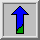# Logical AND/NAND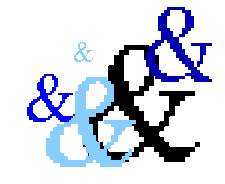Common Names: AND, NAND

## Brief Description

AND and NAND are examples of logical operators having thetruth-tables shown in Figure 1.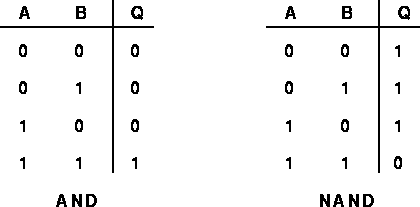Figure 1 Truth-tables for AND and NAND.

As can be seen, the output values of NAND are simply the inverse of the corresponding output values of AND.

The AND (and similarly the NAND) operator typically takes two binary or integer graylevel images as input, and outputs a third image whose pixel values are just those of the first image, ANDed with the corresponding pixels from the second. A variation of this operator takes just a single input image and ANDs each pixel with a specified constant value in order to produce the output.

## How It Works

The operation is performed straightforwardly in a single pass. It is important that all the input pixel values being operated on have the same number of bits in them or unexpected things may happen. Where the pixel values in the input images are not simple 1-bit numbers, the AND operation is normally (but not always) carried out individually on each corresponding bit in the pixel values, in bitwise fashion.## Guidelines for Use

The most obvious application of AND is to compute the intersection of two images. We illustrate this with an example where we want to detect those objects in a scene which did not move between two images, i.e. which are at the same pixel positions in the first and the second image. We illustrate this example using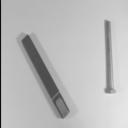and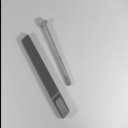If we simply AND the two graylevel images in a bitwise fashion we obtain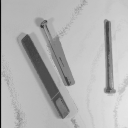Although we wanted the moved object to disappear from the resulting image, it appears twice, at its old and at its new position. The reason is that the object has rather low pixel values (similar to a logical 0) whereas the background has a high values (similar to a logical 1). However, we normally associate an object with logical 1 and the background with logical 0, therefore we actually ANDed the negatives of two images, which is equivalent to NOR them. To obtain the desired result we have to invert the images before ANDing them, as it was done in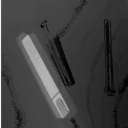Now, only the object which has the same position in both images is highlighted. However, ANDing two graylevel images might still cause problems, as it is not guaranteed that ANDing two high pixel values in a bitwise fashion yields a high output value (for example, 128 AND 127 yields 0). To avoid these problems, it is best to produce a binary versions from the grayscale images using thresholding.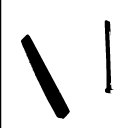and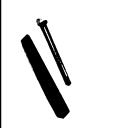are the thresholded versions of the above images andis the result of ANDing their negatives.

Although ANDing worked well for the above example, it runs into problems in a scene like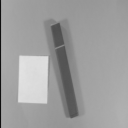Here, we have two objects with the average intensity of one being higher than the background and the other being lower. Hence, we can't produce a binary image containing both objects using simple thresholding. As can be seen in the following images, ANDing the grayscale images is not successful either. If in the second scene the light part was moved, as in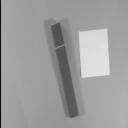then the result of ANDing the two images is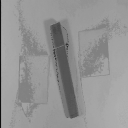It shows the desired effect of attenuating the moved object. However, if the second scene is somehow like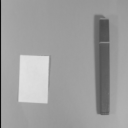where the dark object was moved, we obtain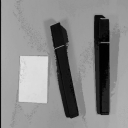Here, the old and the new positions of the dark object are visible.

In general, applying the AND operator (or other logical operators) to two images in order to detect differences or similarities between them is most appropriate if they are binary or can be converted into binary format using thresholding.

As with other logical operators, AND and NAND are often used as sub-components of more complex image processing tasks. One of the common uses for AND is for masking. For example, suppose we wish to selectively brighten a small region of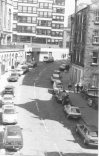to highlight a particular car. There are many ways of doing this and we illustrate just one. First a paint program is used to identify the region to be highlighted. In this case we set the region to black as shown in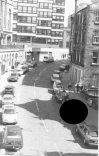This image can then be thresholded to just select the black region, producing the mask shown inThe mask image has a pixel value of 255 (11111111 binary) in the region that we are interested in, and zero pixels (00000000 binary) elsewhere. This mask is then bitwise ANDed with the original image to just select out the region that will be highlighted. This producesFinally, we brighten this image by scaling it by a factor of 1.1, dim the original image using a scale factor of 0.8, and then add the two images together to produce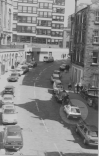AND can also be used to perform so calledbit-slicing on an 8-bit image. To determine the influence of one particular bit on an image, it is ANDed in a bitwise fashion with a constant number, where the relevant bit is set to 1 and the remaining 7 bits are set to 0. For example, to obtain thebit-plane 8 (corresponding to the most significant bit) of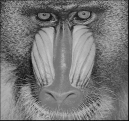we AND the image with 128 (10000000 binary) and threshold the output at a pixel value of 1. The result, shown in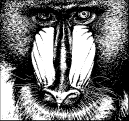is equivalent to thresholding the image at a value of 128. Images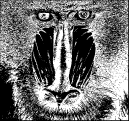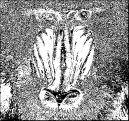and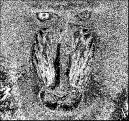correspond to bit-planes 7, 6 and 4. The images show that most image information is contained in the higher (more significant) bits, whereas the less significant bits contain some of the finer details and noise. The image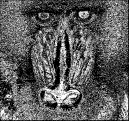shows bit-plane 1.

## Interactive Experimentation

You can interactively experiment with this operator by clicking here.

## Exercises

1. NANDand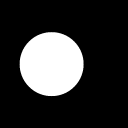Compare the result with the result of ANDing the negatives of the two input images.

2. ANDandas well as the negatives ofandCompare the results with the ones obtained in the previous section.

3. Extract all 8 bit planes from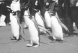and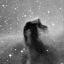Comment on the number of visually significant bits in each image.

4. What would be the effect of ANDing an 8-bit graylevel image with a constant value of 240 (11110000 in binary)? Why might you want to do this?

5. What would be the effect of ANDing an 8-bit graylevel image with a constant value of 15 (00001111 in binary)? Why might you want to do this? Try this out on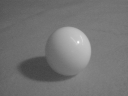and comment on what you see.

## References

E. Davies Machine Vision: Theory, Algorithms and Practicalities, Academic Press, 1990, Chap. 2.

R. Gonzalez and R. Woods Digital Image Processing, Addison-Wesley Publishing Company, 1992, pp 47 - 51, 171 - 172.

A. Jain Fundamentals of Digital Processing, Prentice Hall, 1989, pp 239 - 240.

B. Horn Robot Vision, MIT Press, 1986, pp 47 - 48.

## Local Information